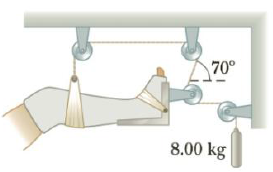Chapter 4, Problem 53P

Chapter
Section
Textbook Problem

A setup similar to the one shown in Figure P4.53 is often used in hospitals to support and apply a traction force to an injured leg. (a) Determine the force of tension in the rope supporting the leg. (b) What is the traction force exerted on the leg? Assume the traction force is horizontal.Figure P4.53

(a)

To determine
The tension on the rope.

Explanation

Given Info: Mass attached is 8.0 kg.

The free body diagram is given below.

The tension (T) equals the weight.

T=mg (I)

• m is the mass attached.
• g is the acceleration due to gravity.

Substitute 8.0 kg for m and 9.8ms2 for g in Equation (I) to get T.

T=(8

(b)

To determine
The traction force.

Still sussing out bartleby?

Check out a sample textbook solution.

See a sample solution

The Solution to Your Study Problems

Bartleby provides explanations to thousands of textbook problems written by our experts, many with advanced degrees!

Get Started